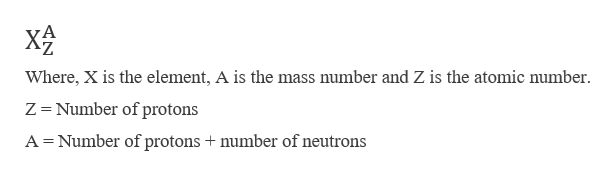Write the atomic symbol for each of the followinga. an atom with 47 protons and mass number of 107b. an atom with 74 protons and 110 neutrons

Question

Write the atomic symbol for each of the following

a. an atom with 47 protons and mass number of 107

b. an atom with 74 protons and 110 neutrons

Step 1

The atomic symbol for each of the following are,

The representation of any atom is given by its atomic number and mass number. The general symbol is represented as,help_outlineImage TranscriptioncloseWhere, X is the element, A is the mass number and Z is the atomic number Z =Number of protons A Number of protons + number of neutrons fullscreen
Step 2
1. Atom with 47 protons and mass number of 107

Z = 47

A = 107

The atomic number of 47 and mass number of 107 co...

Want to see the full answer?

See Solution

Want to see this answer and more?

Our solutions are written by experts, many with advanced degrees, and available 24/7

See Solution
Tagged in

General Chemistry